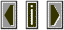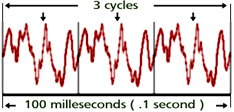PeriodThe time required for a repeating sound to complete one cycle is known as the period of the wave. This is usually expressed in seconds per cycle and can be easily calculated using the following equation:
Period = 1 / frequencyTo determine the period for the 30 Hertz tone shown above we evaluate the following equation:
1 / 30 hertz = 0.033 seconds